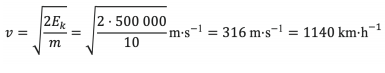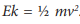# Practical Exercise 3: Positional and Kinetic Energy

Practical Exercise: The ten-kilogram weight is at rest at a height of 10 km above the Earth's surface. Calculate the positional energy according to the equation Ep = mhg, kde g = 10 N·kg–1, h = 5 km. How much energy is converted from positional energy to kinetic energy if the body is moved from 10 km to 5 km? Estimate what maximum speed the weight can reach if we do not take air resistance into account?

Answer: Positional energy Ep = mhg = 10 ∙ 5 000 ∙ 10 J = 500 000 J. All previously calculated positional energy is converted into kinetic energy (the law of conservation of mechanical energy applies). This equation applies to kinetic energy Ek = ½ mv2 . From this formula we express the speed.### Impact on Earth

Practical Exercise: Let's look at the energy that is released when a moving object suddenly stops – e.g. a comet or minor planet hits the Earth. The usual velocities of minor planets hitting the Earth range from 20 km·s–1 do 70 km·s–1.

Imagine a kilogram object that hits the Earth at speed 20 km·s–1. Calculate how much energy is released during this collision.

Answer: This equation applies to kinetic energyWe substitute in base units, so the speed needs to be converted to m·s–1.By calculation we find that= ½ · 1 · (20 000)2 J = 2 ∙ 108 J. Just to give you an idea, this energy would be enough to heat 500 litres of water from 0 °C to 95 °C.

Practical Exercise: Now, imagine the same object, only hitting the Earth at a speed of 70 km · s–1. Calculate how much energy is released during this collision. Compare with the previous value.

Answer: By calculation we find that= ½ · 1 · (70 000)2 J = 2,5 ∙ 109 J. Although the speed increased 3.5 times, the energy increased 2,5 ∙ 109 : 2 ∙ 108 = 12,5-krát.

Practical Exercise: We will look at the effect of the size of the incident object on the released energy. Calculate the released energy of a two-kilogram object that collides with the Earth at speed 20 km · s–1. Compare with the answer in the first case.

Answer: By calculation we find that= ½ · 2 · (20 000)2 J = 4 ∙ 108 J. When the weight of an object doubles, the energy released also doubles.